Basic Geo A - Activity 4 Quiz
Identify *
1 point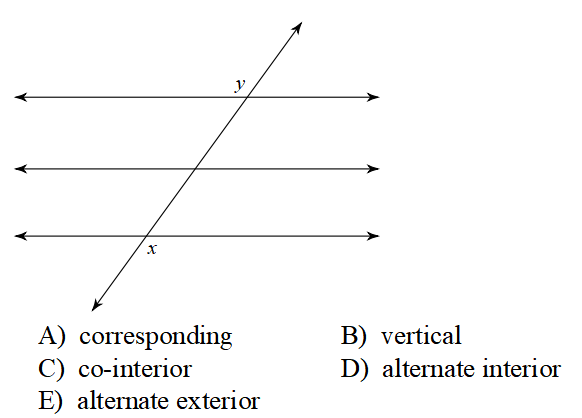Identify the relationship of these two angles. *
1 point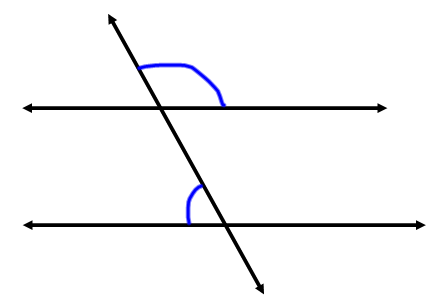Period *
These angles are... *
2 points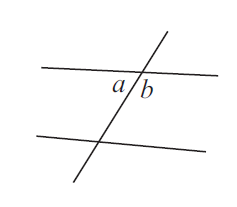What's the relationship between <m and <r? *
1 point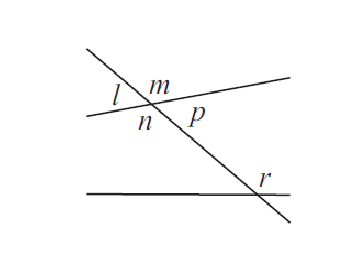Which option MUST BE TRUE?!?!?! *
1 point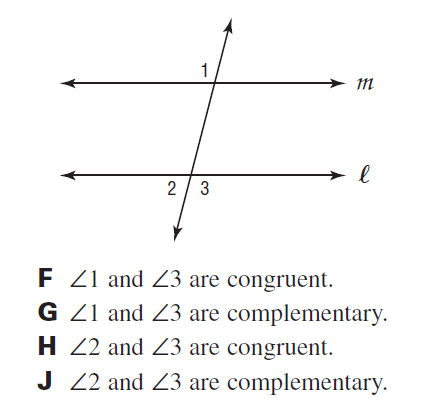find x *
1 point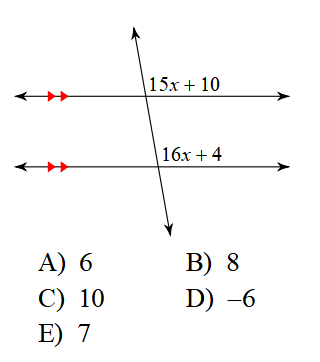Find x *
1 point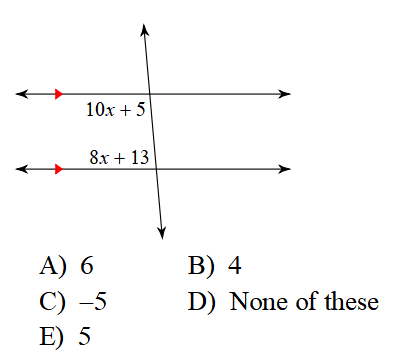Find the missing angle *
2 points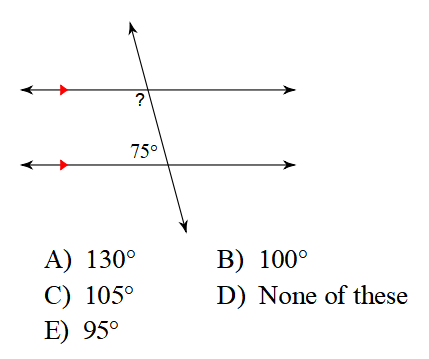What is the relationship between <r and <n? *
1 point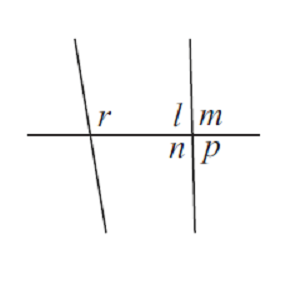Find the value for y. *
1 point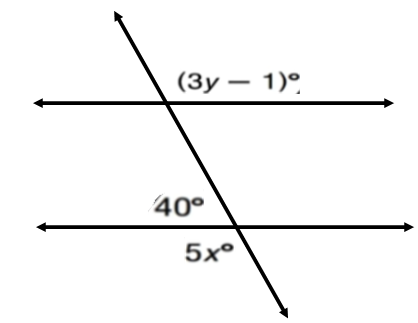Last Name *
Find the missing angle *
2 points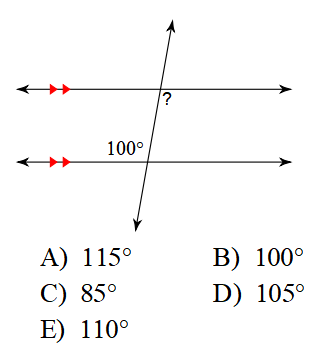Find the measure of angle b only. *
1 point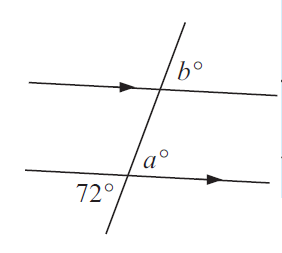Find x. *
1 point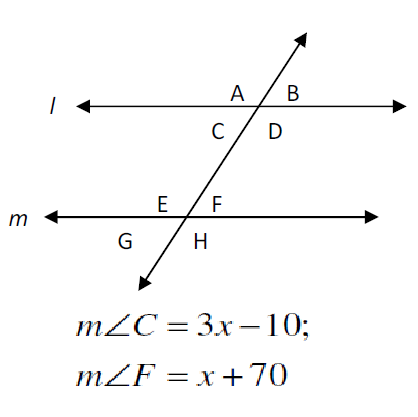Find x. *
2 points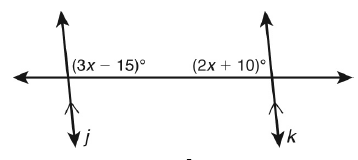Find angle s. *
2 pointsNever submit passwords through Google Forms.
This content is neither created nor endorsed by Google. Report Abuse - Terms of Service - Privacy Policy